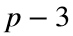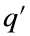# Problem 46046. Determine whether a number is a cluster prime

A cluster prime is an odd prime number p such that all even numbers less than or equal tocan be expressed as a difference of two primes q andthat are no larger than p.
Write a function to determine whether a number is a cluster prime.

### Solution Stats

54.29% Correct | 45.71% Incorrect
Last Solution submitted on Apr 27, 2023

### Community Treasure Hunt

Find the treasures in MATLAB Central and discover how the community can help you!

Start Hunting!